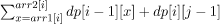# Count of non-decreasing Arrays arr3[] such that arr1[i] <= arr3[i] <= arr2[i]

## Introduction

This blog will discuss the solution to the problem to get count of non-decreasing arrays arr3[] such that arr1[i] <= arr3[i] <= arr2[i].

### Problem Statement

We are given two arrays sorted arrays that are having the same size N. We have to count all the non-decreasing arrays arr3[] of size N such that this condition (arr1[i] <= arr3[i] <= arr2[i]) holds true for every i in the range [0, N].

So before we deep dive into the solution to this problem, we should first look at some examples to better understand the problem.

### Sample Examples

Example 1:

``````Input:
arr1[]={1, 2}
arr2[]={2, 4}

Output:
6

Explanation:
The 6 possible arrays that follows the given conditions {1, 2}, {1, 3}, {1, 4}, {2, 2}, {2, 3}, {2, 4} ``````

Example 2:

``````Input:
arr1[]={2, 5}
arr2[]={3, 8}

Output:
8

Explanation:
The 8 possible arrays that follows the given conditions {2, 5}, {2, 6}, {2, 7}, {2, 8}, {3, 5}, {3, 6}, {3, 7}, {3, 8} ``````

## Dynamic Programming Approach

To solve this problem (count of non-decreasing Arrays arr3[] such that arr1[i] <= arr3[i] <= arr2[i]), we can use dynamic programming. We will make a 2d-array dp[][] such that dp[i][j] will represent the count of arrays of length i such that ith element is j. We will intialize all the elements of the dp array as 0 and we will intialize dp=1

The dp relation can be stated as:

dp[i][j] =By using the above relation, we will calculate the value of dp[i][j] for each i in the range of [0, N] and for each j in the range [0, S], where S will represent the maximum integer in both of the given arrays. The answer will be stored in dp[N][S].

### Steps of Algorithm

1. First,  we will make a 2d-array dp[][] such that dp[i][j] will represent the count of arrays of length i such that ith element is j.

2. After that, we will initialize all the elements of the dp array as 0 and we will initialize dp=1.

dp[i][j] =3. We will use the above relation, to calculate the value of dp[i][j] for each i in the range of [0, N] and for each j in the range [0, S], where S will represent the maximum integer in both of the given arrays.

4. The answer would be stored in dp[N][S].

### Implementation in C++

``````// C++ Program for the problem get count of non-decreasing Arrays arr3[] such that arr1[i] <= arr3[i] <= arr2[i]
#include <bits/stdc++.h>
using namespace std;

// to find count
int arrCount(int arr1[], int arr2[], int N)
{

// max possible value of arr1 and arr2
int S = 1000;

// to store the dp states
vector<vector < int>> dp(N + 1, vector<int> (S + 1, 0));

// initial condition
dp = 1;

// to iterate over the range[0, N]
for (int i = 0; i <= N; i++)
{

// to iterate over the range[0, M]
for (int j = 0; j < M; j++)
{
dp[i][j + 1] += dp[i][j];
}

// if the current index is not equal to the final index
if (i != N)
{
// to iterate over the range[arr1[i], arr2[i]]
for (int j = arr1[i]; j <= arr2[i]; j++)
dp[i + 1][j] += dp[i][j];
}
}

return dp[N][M];
}

// Driver Code for the problem get count of non-decreasing Arrays arr3[] such that arr1[i] <= arr3[i] <= arr2[i]
int main()
{
int arr1[] = { 2, 5 };
int arr2[] = { 3, 8 };

int N = sizeof(arr1) / sizeof(int);

cout << arrCount(arr1, arr2, N);

return 0;
}``````

Output:

``````Input: {2, 5}
{3, 8}

Output: 8``````

#### Complexity Analysis

Time Complexity: O(N*S)

Here S represents the maximum value of the elements of both the arrays.

Space Complexity: O(N*S)

Q1. What is space complexity?

Ans. The space complexity of an algorithm is the overall space taken by algorithm w.r.t input size.

Q2. What is time complexity?

Ans. The time complexity of an algorithm can be defined as the time taken by the computer to run an algorithm.

Q3. What is dynamic programming?

Ans. Dynamic programming is a technique where the problem is broken into sub-problems so that their results can be reused to obtain the optimal solution.

## Key takeaways

In this article, we discussed the problem to get count of non-decreasing arrays arr3[] such that arr1[i] <= arr3[i] <= arr2[i]. We hope you have gained a better understanding of the solution to this problem and, now it is your responsibility to solve the problem and try some new and different approaches to this problem.

Until then, Keep Learning and Keep Coding and practicing on Code studio.